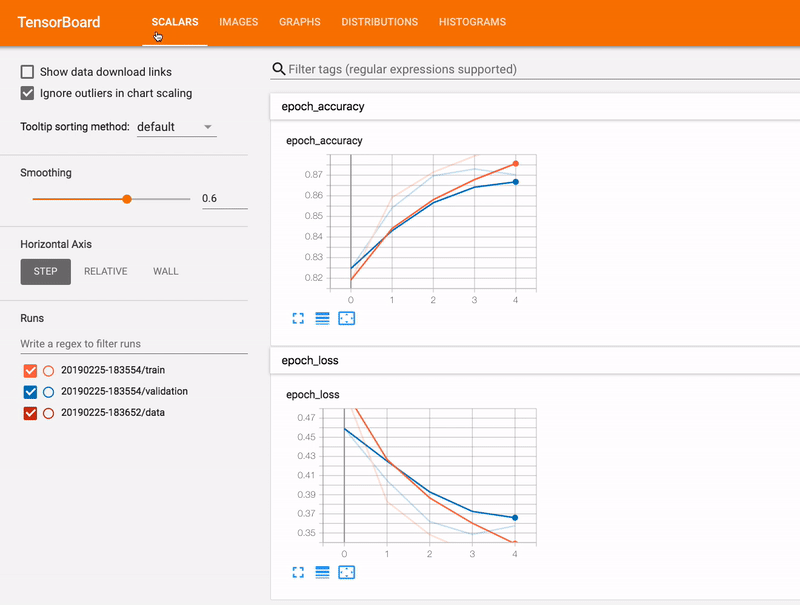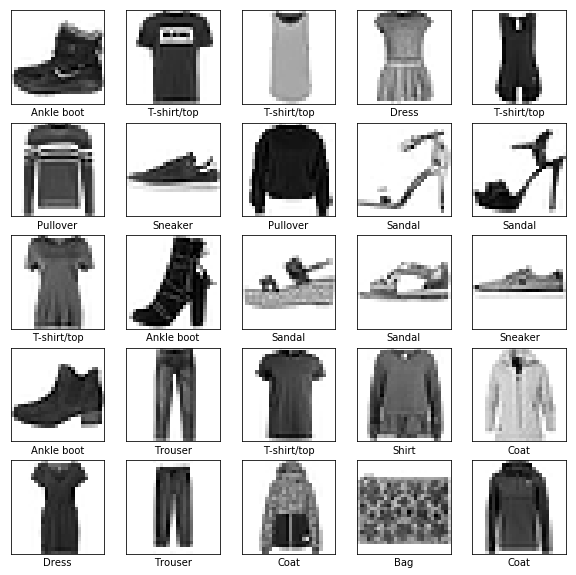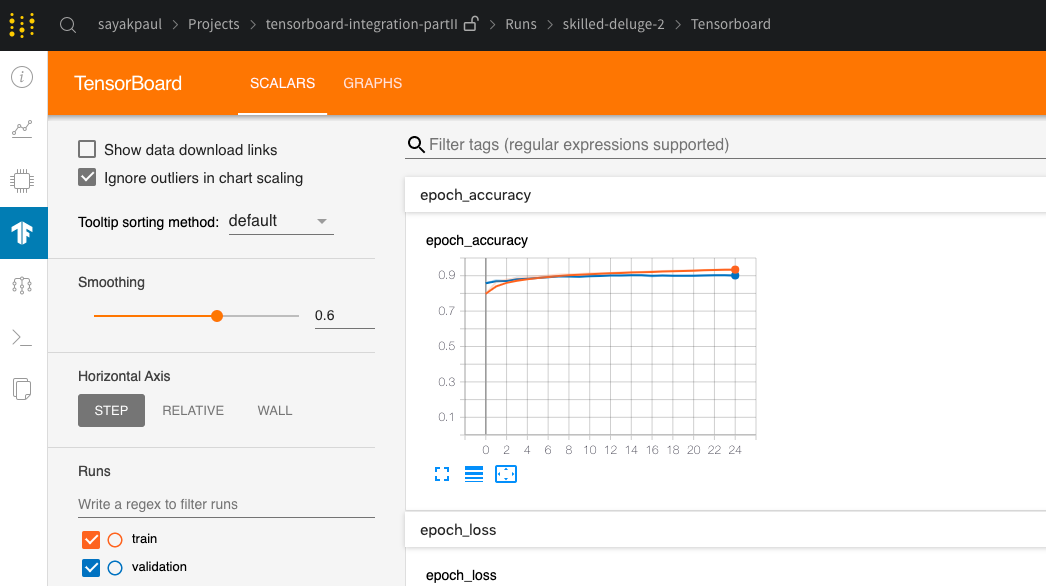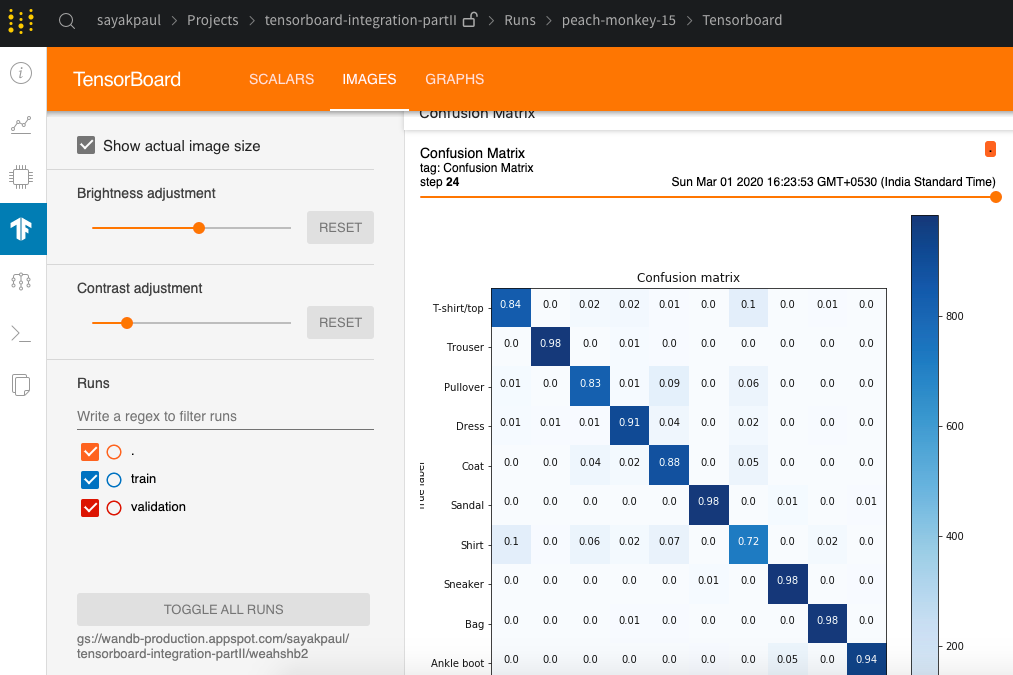‍TensorBoard is a tool for visualizing machine learning models. The model’s performance metrics, parameters, computational graph – TensorBoard enables you to log all of those (and much more) through a very nice web interface.

In this article, we are going to see how to spin up and host a TensorBoard instance online with Weights and Biases. We'll end with visualizing a confusion matrix in TensorBoard.

### Run the Google Colab Notebook →## The Model

To use TensorBoard we first need a model, preferably a one that is easily compatible with TensorBoard. tf.keras makes it very easier to plug TensorBoard in as a callback. We will see how in a moment. But first, let’s build a very simple model and train it to have some data to fill in our TensorBoard instance.

We can create one quickly like so -

``````model = Sequential()
input_shape=(28, 28, 1)))
We will be using the good old FashionMNIST dataset for training this model. Below is a sneak peek of the dataset in case you have not worked with the dataset before:
``````

## The Dataset

We'll use a good old FashionMNIST dataset for training this model.The dataset consists of images of different apparels and each image is 28x28 pixels. The images are grayscale images. This dataset comes with tf.keras.datasets. We will first be loading it and then will normalize the pixel values to the range of [0,1].

``````# Load and preprocess the data
(X_train, y_train), (X_test, y_test) = fashion_mnist.load_data()
X_train /= 255.
X_test /= 255.
``````

Now that we have set up our model and made our data ready to go into our model, let’s move onto model training. We first need to initialize W&B with `sync_tensorboard = True` to sync the event files for a hosted TensorBoard environment.

``````wandb.init(project="your-project-name", sync_tensorboard=True)
``````

Now, in the model training step, we simply need to pass the TensorBoard callback with an appropriate `log_dir` argument. In this case, that value should be wandb.run.dir.

``````model.fit(X_train, y_train, validation_data=(X_test, y_test), epochs=config.epochs,
callbacks=[WandbCallback(data_type="image", labels=labels), TensorBoard(log_dir=wandb.run.dir)])
``````

Once you run the model training step, on your W&B run page (which resembles `app.wandb.ai/<username>/<project-name>/runs/<run-name>`), you should see the TensorBoard.

## Explore a live TensorBoard →We can log a confusion matrix for our model in an epoch-wise manner in a TensorBoard instance. Confusion matrices help us in understanding the model - for instance, we can explore where the model is making incorrect predictions, and which classes it has the hardest time with. This is particularly helpful to do during the model training because it gives us an insight into whether the model is improving at its predictions through its training.

## Explore a live confusion matrix →

(Click on `Images` to see the confusion matrix.)That’s all for this article! I cannot wait to see your interesting model visualizations through TensorBoard and Weights and Biases.